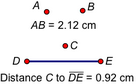# DistanceSelection prerequisites: One of the following combinations of point(s) and straight object(s):

Two points

One or more points and one straight object

One point and one or more straight objects

This Measure menu command measures the distance between two points, or the distance from a point to a straight object, using the distance units chosen on the Units panel of the Preferences dialog box.

Select several points and one straight object to measure the distance from each of the points to the straight object.

Select one point and several straight objects to measure the distance from the point to each of the straight objects.

The distance from a point to a line is the shortest distance from the point to the line and is measured along the perpendicular. The distance from a point to a ray or segment is defined to be the same as the distance from the point to the straight line that contains the ray or segment.

To measure the distance between two points on a coordinate system in grid units, use the Coordinate Distance measurement instead.